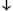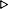ToC DocOverview CGDoc RelNotes Index PermutedIndex
Allegro CLANSI Common Lisp6 Iteration6.2 Dictionary of Iteration

 6.2.4 loop Macro

Syntax:
The "simple" loop form:

loop {compound-form}*{result}*

The "extended" loop form:

loop [name-clause] {variable-clause}* {main-clause}*{result}*

name-clause::= named name

variable-clause::=with-clause |initial-final |for-as-clause

with-clause::= with var1 [type-spec] [= form1] {and var2 [type-spec] [= form2]}*

main-clause::=unconditional |accumulation |conditional |termination-test |initial-final

initial-final::= initially {compound-form}+ | finally {compound-form}+

unconditional::= {do | doing} {compound-form}+ | return {form | it}

accumulation::=list-accumulation |numeric-accumulation

list-accumulation::= {collect | collecting | append | appending | nconc | nconcing} {form | it}
[into simple-var]

numeric-accumulation::= {count | counting | sum | summing |
maximize | maximizing | minimize | minimizing} {form | it}
[into simple-var] [type-spec]

conditional::= {if | when | unless} formselectable-clause {andselectable-clause}*
[elseselectable-clause {andselectable-clause}*]
[end]

selectable-clause::=unconditional |accumulation |conditional

termination-test::= while form | until form | repeat form | always form | never form | thereis form

for-as-clause::= {for | as}for-as-subclause {andfor-as-subclause}*

for-as-subclause::=for-as-arithmetic |for-as-in-list |for-as-on-list |for-as-equals-then |for-as-across |for-as-hash |for-as-package

for-as-arithmetic::= var [type-spec]for-as-arithmetic-subclause

for-as-arithmetic-subclause::=arithmetic-up |arithmetic-downto |arithmetic-downfrom

arithmetic-up::= [[{from | upfrom} form1 |   {to | upto | below} form2 |   by form3]]+

arithmetic-downto::= [[{from form1} |   {{downto | above} form2} |   by form3]]

arithmetic-downfrom::= [[{downfrom form1} |   {to | downto | above} form2 |   by form3]]

for-as-in-list::= var [type-spec] in form1 [by step-fun]

for-as-on-list::= var [type-spec] on form1 [by step-fun]

for-as-equals-then::= var [type-spec] = form1 [then form2]

for-as-across::= var [type-spec] across vector

for-as-hash::= var [type-spec] being {each | the}
{{hash-key | hash-keys} {in | of} hash-table
[using (hash-value other-var)] |
{hash-value | hash-values} {in | of} hash-table
[using (hash-key other-var)]}

for-as-package::= var [type-spec] being {each | the}
{symbol | symbols |
present-symbol | present-symbols |
external-symbol | external-symbols}
[{in | of} package]

type-spec::=simple-type-spec |destructured-type-spec

simple-type-spec::= fixnum | float | t | nil

destructured-type-spec::= of-type d-type-spec

d-type-spec::= type-specifier | (d-type-spec . d-type-spec)

var::=d-var-spec

var1::=d-var-spec

var2::=d-var-spec

other-var::=d-var-spec

d-var-spec::= simple-var | nil | (d-var-spec .d-var-spec)

Arguments and Values:
compound-form - a compound form.

name - a symbol.

simple-var - a symbol (a variable name).

form, form1, form2, form3 - a form.

step-fun - a form that evaluates to a function of one argument.

vector - a form that evaluates to a vector.

hash-table - a form that evaluates to a hash table.

package - a form that evaluates to a package designator.

type-specifier - a type specifier. This might be either an atomic type specifier or a compound type specifier, which introduces some additional complications to proper parsing in the face of destructuring; for further information, see Section 6.1.1.7 Destructuring.

result - an object.

Description:
For details, see Section 6.1 The LOOP Facility.

Examples:
```;; An example of the simple form of LOOP.
(loop (format t "~&Number: ")
(let ((n (parse-integer (read-line) :junk-allowed t)))
(when (not n) (return))
(format t "~&The square root of ~D is ~D.~%" n (sqrt n)))))SQRT-ADVISORNumber: 5[Return]The square root of 5 is 2.236068.Number: 4[Return]The square root of 4 is 2.Number: done[Return]NIL

;; An example of the extended form of LOOP.
(loop as n = (progn (format t "~&Number: ")

while n
do (format t "~&The square of ~D is ~D.~%" n (* n n))))SQUARE-ADVISORNumber: 4[Return]The square of 4 is 16.Number: 23[Return]The square of 23 is 529.Number: done[Return]NIL

;; Another example of the extended form of LOOP.
(loop for n from 1 to 10
when (oddp n)
collect n)(1 3 5 7 9)
```

do, dolist, dotimes, return, go, throw, Section 6.1.1.7 Destructuring

Notes:
Except that loop-finish cannot be used within a simple loop form, a simple loop form is related to an extended loop form in the following way:

``` (loop {compound-form}*) ==(loop do {compound-form}*)
```

Allegro CL Implementation Details:

See cl:loop and the for-as-in-sequnce subclause for looping over sequences for a description of the additional for-as-in-sequence clause in Allegro CL. Note the links are to the documentation for the current Allegro CL version. Replace /current/ in the URL with the Allegro CL version number to see similar documentation in earlier releases.© Franz Inc. 1998-2019 - File last updated 02-07-2019